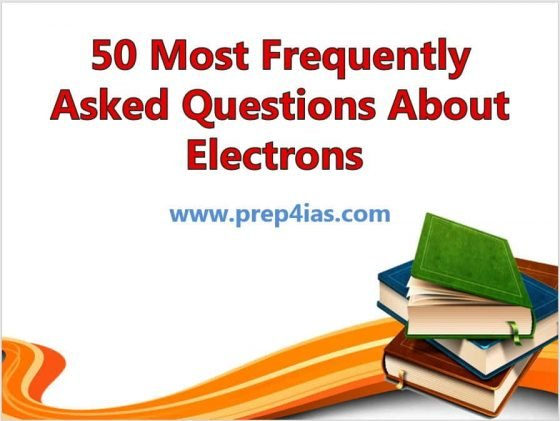In this article, I will take you through 50 Most Frequently Asked Questions About Electrons that every learner must be aware of especially the ones who is preparing for Competitive exams. Electrons belong to the first generation of the lepton particle family, and are generally thought to be elementary particles because they have no known components or substructure. The electron has a mass that is approximately 1/1836 that of the proton. We will see some of the most frequently asked questions about electron in below section. More on wikipedia.### 1. What is Electron ?

Ans. An electron is a stable subatomic particle whose electric charge is negative one elementary charge.

### 2. How many valence electrons does Carbon have ?

Ans. 4 valence electrons

Ans. 6 electrons

### 4. How many valence electrons does Oxygen have ?

Ans. 6 valence electrons

Ans. 8 electrons

### 6. How many electrons does Platinum have ?

Ans. 78 electrons

### 7. How many valence electrons does Nitrogen have ?

Ans. 5 valence electrons

### 8. How many valence electrons does Chlorine have ?

Ans. 7 valence electrons

### 9. How many valence electrons does Sodium have ?

Ans. 1 valence electron

### 10. How many valence electrons does Sulfur have ?

Ans. 6 valence electrons

### 11. What is the charge of an Electron ?

Ans. one elementary charge of an electron is equals to 1.60217662 × 10^-19 coulombs.

Ans. J.J Thomson

### 13. What are Valence Electrons ?

Ans. Valence electrons are the electrons which are located on the outermost shell of an atom.

### 14. How many Valence electrons does Aluminum have ?

Ans. 3 valence electrons

### 15. How many Valence electrons does Calcium have ?

Ans. 2 valence electrons

### 16. How many Valence electrons does Fluorine have ?

Ans. 7 valence electrons

### 17. How many Valence electrons does Hydrogen have ?

Ans. 1 valence electron

### 18. How many Valence electrons does Magnesium have ?

Ans. 2 valence electrons

### 19. How many Valence electrons does Phosphorous have ?

Ans. 5 valence electrons

### 20. How to find the number of electrons in a shell ?

Ans. The maximum number of electrons present in a particular shell is calculated by the formula 2n^2, where "n" represents the shell number.

### 21. What bond is formed when electrons are shared ?

Ans. Covalent Bond

### 22. Where are electrons located in an Atom ?

Ans. Electrons are located in the nucleus of an Atom.

Ans. 7 electrons

### 24. How many electrons does Sodium have ?

Ans. 11 electrons

### 25. How many valence electrons does Boron have ?

Ans. 3 valence electrons

### 26. How many valence electrons does Helium have ?

Ans. 2 valence electrons

### 27. How many valence electrons does Lithium have ?

Ans. 1 valence electron

### 28. How many valence electrons does Potassium have ?

Ans. 1 valence electron

### 29. How many valence electrons does Silica have ?

Ans. 4 valence electrons

### 30. How many electrons does Tungsten have ?

Ans. 74 electrons

Ans. 1 electron

Ans. 3 electrons

### 33. How many electrons does Neon have ?

Ans. 10 electrons

### 34. How many valence electrons does Beryllium have ?

Ans. 2 valence electrons

### 35. How many valence electrons does Bromine have ?

Ans. 7 valence electrons

### 36. How many valence electrons does Iodine have ?

Ans. 7 valence electrons

### 37. What is Electron Affinity ?

Ans. The amount of energy released after an electron is added to a neutral atom to form a negatively charged ion.

### 38. Why are valence electrons important ?

Ans. Valence electrons are the one which takes part in a chemical reaction. The number of valence electrons determines the reactivity of a specific element. The number of electrons in atom that actually take part in a chemical reaction is known as the valency of the element.

### 39. Are electrons positive or negative ?

Ans. An electron carries a negative charge.

### 40. How many electrons does Aluminum have ?

Ans. 13 electrons

### 41. What is the mass of an electron ?

Ans. An electron mass is equal to 9.10938356 × 10^-31 kilograms.

### 42. Where are electrons located in an Atom ?

Ans. Electrons exist in a cloud orbiting the nucleus in an atom.

### 43. Do Isotopes have the same number of Electrons ?

Ans. Yes isotopes will have the same number of electrons.

Ans. 8 electrons

Ans. Positron

### 46. Do electrons and protons attract each other ?

Ans. An electron carries a negative charge and a proton carries a positive charge and we know that opposite charges attract each other so electrons and protons attract each other.

### 47. Who named the charge as electron in 1891 ?

Ans. George Johnstone Stoney

### 48. Does electrons exhibit the properties of particles or waves ?

Ans. Electrons exhibit the properties of both particles and waves.

### 49. How many valence electrons does Arsenic have ?

Ans. 5 valence electrons

### 50. How many valence electrons does Zinc have ?

Ans. 2 valence electrons